# Cylindrical Roof Under Its Own WeightThis test, also known as the Scordelis-Lo roof, lets you check analysis results for a cylindrical roof under its own weight, in the context of a static case. You will use 2D meshes.Reference:

SCORDELIS A.C., LO K.S., Computer Analysis of Cylindrical Shells, Journal of the American Concrete Institute Vol. 61, pp539-561, 1969.

##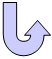Specifications

### Geometry Specifications

 Length: L = 3000 mm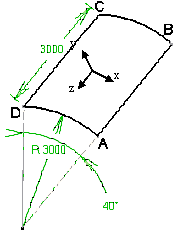Radius: R = 3000 mm Thickness: th = 30 mm Angle: Ø = 40 deg

### Analysis Specifications

 Young Modulus (material): E = 30000 MPa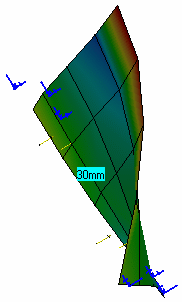Poisson's Ratio (material): ν = 0 Mesh Specifications: Number of nodes: 5 x 5 Number of nodes: 9 x 9 Number of nodes: 15 x 15 Restraints (User-defined): On B-C: Tx = Ty = Rz = 0 On C-D: Tz = Rx =Ry = 0 On D-A: Tz = Rx = Rz = 0 Loads (surface force density = weight of the roof): q = 6250 N_m 2 on y

##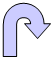Results

The results (in mm) correspond to the vertical displacement at Point A.

 Number of Nodes Theoretical Values Computed Values Linear Triangle  (TR3) Parabolic Triangle  (TR6) Linear Quadrangle  (QD4) Parabolic Quadrangle (QD8) Shallow Shell Deep Shell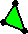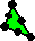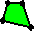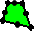5 x 5 37.03 36.10 29.56 40.83 40.18 37.05 9 x 9 37.03 36.10 34.57 37.88 37.84 36.39 15 x 15 37.03 36.10 37.04 38.54 37.12 36.74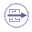To Perform the Test:

The Cylindrical_Roof_QD8_15nodes.CATAnalysis document presents a complete analysis of this case, computed with a mesh formed of parabolic quadrangle elements (QD8).

To compute the case with linear quadrangle (QD4), linear triangle (TR3) and parabolic triangle (TR6) elements, proceed as follow:

1. Open the CATAnalysis document.

2. In the Advanced Meshing Tools workbench, replace the mesh specifications as indicated above.

3. In the Generative Structural Analysis workbench, compute the case.